# Flatten Pixmap VI

## LabVIEW 2018 Help

Edition Date: March 2018
Part Number: 371361R-01
View Product InfoLabVIEW 2016 HelpLabVIEW 2017 HelpLabVIEW 2018 HelpLabVIEW 2019 HelpLabVIEW 2020 Help

Owning Palette: Graphics Formats VIs

Requires: Base Development System

Converts pixmap data from a 2D array to a 1D array.

You can write the resulting 1D array to a file or use the low-level Draw Flattened Pixmap VI to view it.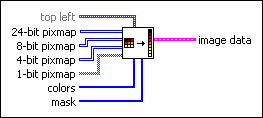top left specifies in coordinates where to place the top-left corner of the image.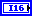x is the horizontal coordinate that increases to the right.y is the vertical coordinate that increases to the bottom.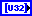24-bit pixmap is the 2D array of data to convert to image data. The dimensions of the image data match the dimensions of this array.8-bit pixmap is the 2D array of data to convert to image data. The dimensions of the image data match the dimensions of this array. The VI uses the data as indexes into the color array.4-bit pixmap is the 2D array of data to convert to image data. The dimensions of the image data match the dimensions of this array. The VI uses the data as indexes into the color array.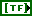1-bit pixmap is the 2D array of data to convert to image data. The dimensions of the image data match the dimensions of this array. Elements that are FALSE map to element 0 in the color table. Elements that are TRUE map to element 1 in the color table.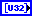colors is an array of RGB color values that correspond to the values in the pixmap input you wire. Which pixmap input you wire determines how LabVIEW interprets the value of this input. If you wire data to 24-bit pixmap, LabVIEW ignores this input. If you wire data to 8-bit pixmap, the array can have 256 elements. If you wire data to 4-bit pixmap, the array can have 16 elements. If you wire data to 1-bit pixmap, the array can have 2 elements. If the colors array is empty for any depth other than 24 bits per pixel, the image uses the default LabVIEW color palette.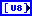mask is a 1D array that describes mask information for each pixel. If the mask array is empty, LabVIEW draws all resulting pixels without transparency.image data returns information about the pixmap so you can use the Draw Flattened Pixmap VI to draw the pixmap as a picture or use the Graphics Formats VIs to save the pixmap to a file.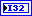image type is reserved for future use.image depth specifies the color depth of the image, which is the number of bits to use to describe the color of each pixel in the image. Valid values include 1, 4, 8, and 24 bits per pixel. image depth affects how LabVIEW interprets the values of image and colors.image is an array of bytes that describes the color of each pixel in the image in raster order. The value of image depth determines how LabVIEW interprets the value of this output.

If image depth is 24, each pixel has three bytes to describe its color. The first byte for each pixel describes the red value, the second byte describes the green value, and the third byte describes the blue value.

If image depth is 8, each pixel has one byte to describe its color. The value of each bit corresponds to an element in colors, which stores 32-bit RGB values where the most significant byte is zero, followed in order by red, green, and blue values. Valid values include 0 through 255.

If image depth is 4, the behavior is similar to when image depth is 8 except valid values in image include 0 through 15.

If image depth is 1, any value of zero in image corresponds to element 0 in colors. All other values correspond to element 1 in colors.

The size of the array might be larger than expected due to padding.mask is an array of bytes in which each bit describes mask information for a pixel. The first byte describes the first eight pixels, the second byte describes the next eight pixels, and so on. If a bit is zero, LabVIEW draws the corresponding pixel as transparent. If the array is empty, LabVIEW draws all pixels without transparency. If the array does not contain a bit for each pixel in the image, LabVIEW draws any pixels missing from the array without transparency.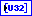colors is an array of RGB color values that correspond to the values in image. The value of image depth determines how LabVIEW interprets the value of this output.

If image depth is 24, LabVIEW ignores this output.

If image depth is 8, the array can have 256 elements.

If image depth is 4, the array can have 16 elements.

If image depth is 1, the array can have 2 elements.

If the array is empty for any depth other than 24 bits per pixel, the image is using the default LabVIEW color palette.Rectangle is a cluster that contains coordinates that describe the bounding rectangle of the image. Horizontal coordinates increase to the right, and vertical coordinates increase to the bottom.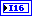left is the horizontal coordinate of the left edge of the rectangle.top is the vertical coordinate of the top edge of the rectangle.right is the horizontal coordinate of the right edge of the rectangle.bottom is the vertical coordinate of the bottom edge of the rectangle.